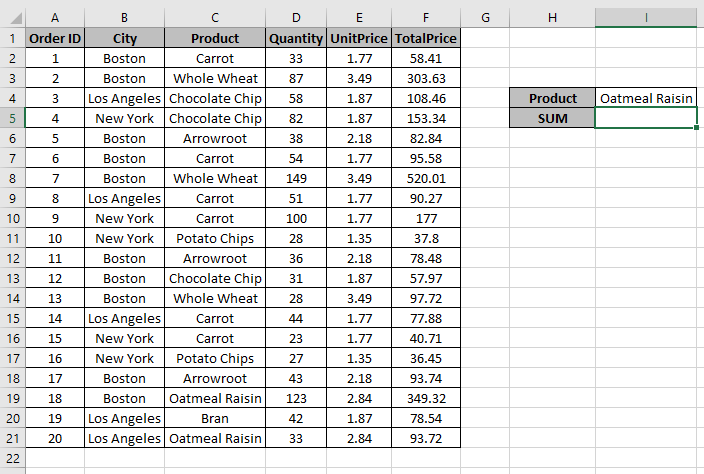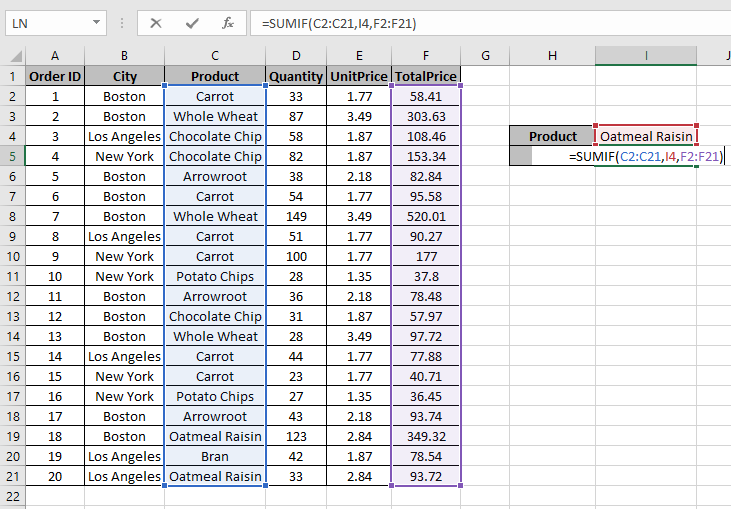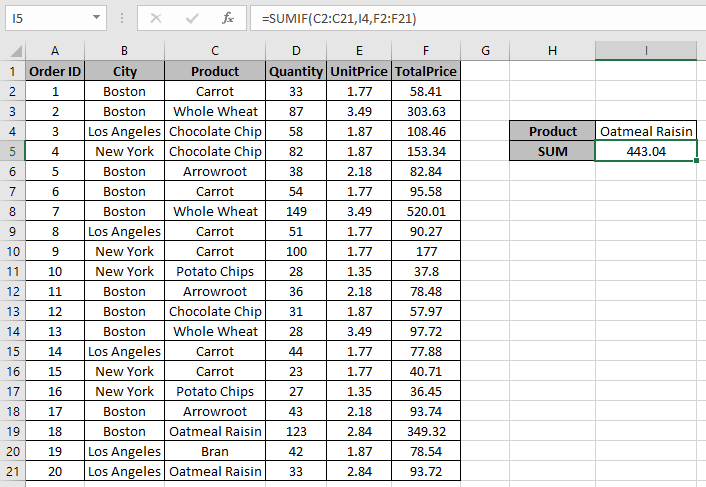# How to Sum if cell contains text in other cell in Excel

In this article, we will learn about how to get the SUM value if cell value is equal to a specific value in other cell in Excel.

In simple words, when working with long data sheets, sometimes we need to extract the sum of values corresponding to where cell is equal to the value in other cell like getting the sum of monthly expense where just a specific day’s (weekday) amount is required.

Let’s get the Generic Formula:

=SUMIF (range, cell reference, sum_range)

range : condition on the array
cell reference : value in referred cell.
sum_range : range where sum is needed

Let’s understand this function using it in an example.Here we have Order IDs from different regions with their respective quantity and Price.

We need to get the sum of Total Price for a specific Product in other cell I4.

Use the formula:

=SUMIF(C2:C21, I4, F2:F21)

Explanation:
C2:C21 : range where formula matches values.
I4 : Value from the other cell to match with C2:C21 range
A2:A26 : sum_range where amount adds up.
=SUMIF({"Carrot";"Whole Wheat";"Chocolate Chip";"Chocolate Chip";"Arrowroot";"Carrot";"Whole Wheat";"Carrot";"Carrot";"Potato Chips";"Arrowroot";"Chocolate Chip";"Whole Wheat";"Carrot";"Carrot";"Potato Chips";"Arrowroot";"Oatmeal Raisin";"Bran";"Oatmeal Raisin"},
I4,
{58.41;303.63;108.46;153.34;82.84;95.58;520.01;90.27;177;37.8;78.48;57.97;97.72;77.88;40.71;36.45;93.74;349.32;78.54;93.72})

This formula adds up all the values corresponding to all the values in cell I4 i.e. “Oatmeal raisinPress Enter to get the SumThe formula returns 443.04 as the sum for the value in other cell.

As you can see this formula returns the sum if cell is equal to a value.

Hope you understood how to get the SUM if cell is equal to value in Excel. Explore more articles on Excel SUMIF function here. Please feel free to state your query or feedback for the above article.

Related Articles:

Popular Articles

50 Excel Shortcut to Increase Your Productivity : Get faster at your task. These 50 shortcuts will make you work even faster on Excel.

How to use the VLOOKUP Function in Excel : This is one of the most used and popular functions of excel that is used to lookup value from different ranges and sheets.

How to use the COUNTIF function in Excel : Count values with conditions using this amazing function. You don't need to filter your data to count specific values. Countif function is essential to prepare your dashboard.

How to use the SUMIF Function in Excel : This is another dashboard essential function. This helps you sum up values on specific conditions.

Terms and Conditions of use

The applications/code on this site are distributed as is and without warranties or liability. In no event shall the owner of the copyrights, or the authors of the applications/code be liable for any loss of profit, any problems or any damage resulting from the use or evaluation of the applications/code.